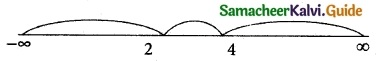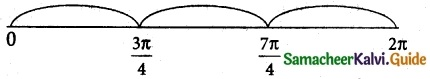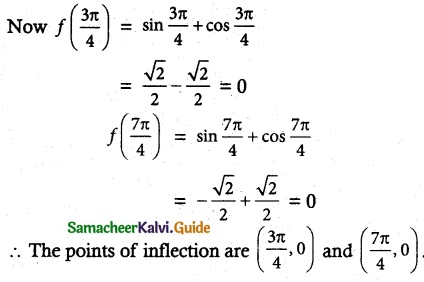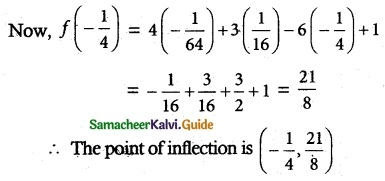Tamilnadu State Board New Syllabus Samacheer Kalvi 12th Maths Guide Pdf Chapter 7 Applications of Differential Calculus Ex 7.7 Textbook Questions and Answers, Notes.

## Tamilnadu Samacheer Kalvi 12th Maths Solutions Chapter 7 Applications of Differential Calculus Ex 7.7

Question 1.
Find intervals of concavity and points of inflection for the following functions:
(i) f(x) = x(x – 4)³
(ii) f(x) = sin x + cos x, 0 < x < 2π
(iii) f(x) = $$\frac { 1 }{ 2 }$$(ex – e-x)
Solution:
(i) f(x) = x(x – 4)³
f'(x) = 3x(x – 4)² + (x – 4)³(1)
= (x – 4)² (4x – 4) = 4 (x – 4)² (x – 1)
f'(x) = 4 [(x-4)² (1) + (x – 1) 2 (x – 4)]
= 4(x – 4)(x – 4 + 2x – 2)
= 4(x – 4)(3x – 6) = 12(x – 4)(x – 2)
f'(x) = 0 ⇒ 12 (x – 4) (x – 2) = 0
Critical points x = 2, 4The intervals are (- ∞, 2), (2, 4) and (4, ∞)
In the interval (-∞, 2), f”(x) > 0 ⇒ Curve is Concave upward
In the interval (2, 4), f”(x) < 0 ⇒ Curve is Concave downward.
In the interval (4, ∞), f”(x) > 0 ⇒ Curve is Concave upward.
The curve is concave upward in
(-∞, 2), (4, ∞) it is concave downward in (2, 4).
f”(x) changes its sign when passing through x = 2 and x = 4
Now f(2) = 2 (2 – 4)³ = -16 and f(4) = 4 (4 – 4)³ = 0
∴ The points of inflection are (2, -16) and (4, 0).(ii) f(x) = sin x + cos x, 0 < x < 2π
f'(x) = cos x – sin x
f”(x) = – sin x – cos x
f'(x) = 0 ⇒ sin x + cos x = 0
Critical points x = $$\frac { 3π }{ 4 }$$, $$\frac { 7π }{ 4 }$$The intervals are (0, $$\frac { 3π }{ 4 }$$), ($$\frac { 3π }{ 4 }$$, $$\frac { 7π }{ 4 }$$) and ($$\frac { 7π }{ 4 }$$, 2π)
In the interval (0, $$\frac { 3π }{ 4 }$$), f'(x) < 0 ⇒ curve is concave down.
In the interval ($$\frac { 3π }{ 4 }$$, $$\frac { 7π }{ 4 }$$), f'(x) > 0 ⇒ curve is concave up.
In the interval ($$\frac { 7π }{ 4 }$$, 2π), f'(x) < 0 ⇒ curve is concave down.
The curve is concave upward in ($$\frac { 3π }{ 4 }$$, $$\frac { 7π }{ 4 }$$) and concave downward in (0, $$\frac { 3π }{ 4 }$$) and ($$\frac { 7π }{ 4 }$$, 2π)
f'(x) changes its sign when passing through x = $$\frac { 3π }{ 4 }$$ and x = $$\frac { 7π }{ 4 }$$(iii) f(x) = $$\frac { 1 }{ 2 }$$ (ex – e-x)
f'(x) = $$\frac { 1 }{ 2 }$$ (ex + e-x)
f”(x) = $$\frac { 1 }{ 2 }$$ (ex – e-x)
f”(x) = 0 ⇒ $$\frac { 1 }{ 2 }$$ (ex – e-x) = 0
Critical point x = 0
The intervals are (-∞, o) and (0, ∞)
In the interval (-∞, 0), f”(x) < 0 ⇒ curve is concave down.
In the interval (0, ∞), f(x) > 0 ⇒ curve is concave up.
∴ The curve is concave up in (0, ∞) and concave down in (-∞, 0).
f'(x) changes its sign when passing through x = 0
Now f(0) = – (e° – e°) = $$\frac { 1 }{ 2 }$$ (1 – 1) = 0
∴ The point of inflection is (0, 0).

Question 2.
Find the local extrema for the following functions using second derivative test:
(i) f(x) = -3x5 + 5x3
(ii) f(x) = x log x
(iii) f(x) = x² e-2x
Solution:
(i) f(x) = – 3x5 + 5x3
f'(x) = 0, f”(x) = -ve at x = a
⇒ x = a is a maximum point
f'(x) = 0, f”(x) = +ve at x = 6
⇒ x = b is a minimum point
f(x) = – 3x5 + 5x3
f’ (x) = -15x4 + 15x2
f”(x) = -60x3 + 30x
f'(x) = 0 ⇒ – 15x2 (x2 – 1) = 0
⇒ x = 0, +1, -1
at x = 0, f”(x) = 0
at x = 1, f”(x) = -60 + 30 = – ve
at x = -1, f”(x) = 60 – 30 = + ve
So at x = 1, f'(x) = 0 and f”(x) = -ve
⇒ x = 1 is a local maximum point.
and f(1) = 2
So the local maximum is (1, 2)
at x = -1, f'(x) = 0 and f”(x) = +ve
⇒ x = -1 is a local maximum point and f(-1) = -2.
So the local minimum point is (-1, -2)
∴ a local minimum is -2 and the local maximum is 2.(ii) f(x) = x log x
f'(x) = x – $$\frac { 1 }{ 4 }$$ + log x = 1 + log x
For maximum or minimum
f'(x) = 0 ⇒ 1 + log x = 0
⇒ log x = -1
x = e-1 = $$\frac { 1 }{ e }$$
f”(x) = $$\frac { 1 }{ x }$$
at x = $$\frac { 1 }{ e }$$, f”(x) > 0 ⇒ f(x) attains minimum.
∴ Local minimum f($$\frac { 1 }{ e }$$) = $$\frac { 1 }{ e }$$ log ($$\frac { 1 }{ e }$$)
= $$\frac { 1 }{ e }$$(-1) = –$$\frac { 1 }{ e }$$

(iii) f(x) = x2 e-2x
f'(x) = x2[-2e-2x] + e-2x (2x)
= 2e-2x (x – x2)
f”(x) = 2e-2x(1 – 2x) + (x – 2) (-4e-2x)
= 2e-2x [(1 – 2x) + (x – x2) (- 2)]
= 2e-2x [2x2 – 4x + 1]
f'(x) = 0 ⇒ 2e-2x(x – x2) = 0
⇒ x (1 – x) = 0
⇒ x = 0 or x = 1
at x = 0, f”(x) = 2 × 1 [0 – 0 + 1] = +ve
⇒ x = 0 is a local minimum point and the minimum value is f(0) = 0 at x = 1,
f”(x) = 2e-2 [2 – 4 + 1] = -ve
⇒ x = 1 is a local maximum point and the maximum value is f(1) = $$\frac{1}{e^{2}}$$
Local maxima $$\frac{1}{e^{2}}$$ and local minima = 0Question 3.
For the function f(x) = 4x³ + 3x² – 6x + 1 find the intervals of monotonicity, local extrema, intervals of concavity and points of inflection.
Solution:
(x) = 4x³ + 3x² – 6x + 1
Monotonicity
f(x) = 4x³ + 3x² – 6x + 1
f'(x) = 12x² + 6x – 6
f'(x) = 0 ⇒ 6(2x² + x – 1) = 0
x = -1, $$\frac { 1 }{ 2 }$$ (Stationary points)
∴ The intervals of monotonicity are (-∞, -1), (-1, $$\frac { 1 }{ 2 }$$) and ($$\frac { 1 }{ 2 }$$, ∞)
In (-∞, -1), f'(x) > 0 ⇒ f(x) is strictly increasing
In (-1, $$\frac { 1 }{ 2 }$$), f'(x) < 0 ⇒ f(x) is strictly decreasing
In ($$\frac { 1 }{ 2 }$$, ∞) f'(x) > 0 ⇒ f(x) is strictly increasing
f(x) attains local maximum as f'(x) changes its sign from positive to negative when passing through x = -1
∴ Local maximum f(-1) = -4 + 3 + 6 + 1 = 6
f(x) attains local minimum as f'(x) changes its sign from negative to positive when passing through x = $$\frac { 1 }{ 2 }$$
∴ Local minimum f($$\frac { 1 }{ 2 }$$)
= 4($$\frac { 1 }{ 8 }$$) + 3($$\frac { 1 }{ 4 }$$) – 6($$\frac { 1 }{ 2 }$$) + 1
= $$\frac { 1 }{ 2 }$$ + $$\frac { 3 }{ 4 }$$ – + 1 = –$$\frac { 3 }{ 4 }$$
f(x) = 4x³ + 3x² – 6x + 1
f'(x) = 12x² + 6x – 6
f”(x) = 24x + 6
f’(x) = 0 ⇒ 24x + 6 = 0
x = –$$\frac { 6 }{ 24 }$$ = –$$\frac { 1 }{ 4 }$$ (critical points)
∴ The intervals are (∞, $$\frac { 1 }{ 4 }$$) and ($$\frac { 1 }{ 4 }$$, ∞) f”(x) > 0
In the interval (-∞, –$$\frac { 1 }{ 4 }$$), f”(x) < 0 ⇒ curve is concave down.
In the interval (-$$\frac { 1 }{ 4 }$$, ∞), f”(x) > 0 ⇒ curve is concave up.
The curve is concave upward in (-$$\frac { 1 }{ 4 }$$, ∞) and concave downward in (-∞, –$$\frac { 1 }{ 4 }$$)
f”(x) changes its sign when passing through x = –$$\frac { 1 }{ 4 }$$